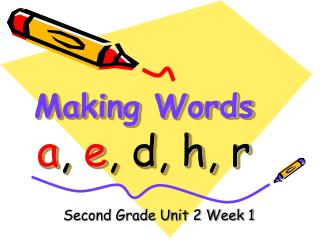DownloadDownload PresentationMaking Words a , e , d, h, r

Making Words a , e , d, h, r

Télécharger la présentationMaking Words a , e , d, h, r

- - - - - - - - - - - - - - - - - - - - - - - - - - - E N D - - - - - - - - - - - - - - - - - - - - - - - - - - -
Presentation Transcript

1. Making Wordsa, e, d, h, r Second Grade Unit 2 Week 1

2. r a e d h h a d had

3. r h a e d r e d red

4. r h a d e e a r ear

5. r h a d e h e a r hear

6. r h a d e d e a r dear

7. h r a e d d a r e dare

8. r d h a e h a r e hare

9. a e d h r r e a d read

10. a e d h r h e a d head

11. a e d h r It’s time for the Secret Word! Spell a word with all the letters. d h r e a

12. Let’s sort some of our words according to their endings.